Register
 Email: Passwd 验 证:换一张 Have you forgotten your password? Stay signed in Log In
 ALL Title Author Keyword Sponsors Type Publication date Submitted Time Subject Conference Name Source Categories KOS Subject Advisor ORCID Advanced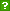ISCAS OpenIR  > 软件所图书馆  > 期刊论文
 Title: Interpolation and Approximation of Sparse Multivariate Polynomials over $GF(2)$ Author: Ron M. Roth ; Gyora M. Benedek Source: SIAM Journal on Computing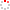Issued Date: 1991 Volume: 20, Issue:2, Pages:291-314 Indexed Type: 其他 Cooperation Status: 其它 English Abstract: A function $f:\{ 0,1\} ^n \to \{ 0,1\}$ is called $t$-sparse if the $n$-variable polynomial representation of $f$ over $GF(2)$ contains at most $t$ monomials. Such functions are uniquely determined by their values at the so-called critical set of all binary $n$-tuples of Hamming weight $\geqq n - \lfloor \log _2 t \rfloor - 1$. An algorithm is presented for interpolating any $t$-sparse function $f$, given the values of $f$ at the critical set. The time complexity of the proposed algorithm is proportional to $n$, $t$, and the size of the critical set. Then, the more general problem of approximating 1-sparse functions is considered, in which case the approximating function may differ from $f$ at a fraction $\varepsilon$ of the space $\{ 0,1\} ^n$. It is shown that $O(({t / \varepsilon }) \cdot n)$ evaluation points are sufficient for the (deterministic) $\varepsilon$-approximation of any $t$-sparse function, and that an order $(t / \varepsilon )^{\alpha (t,\varepsilon )} \cdot \log n$ points are necessary for this purpose, where $\alpha (t,\varepsilon ) \geqq 0.694$ for a large range of $t$ and $\varepsilon$. Similar bounds hold for the $t$-term DNF case as well. Finally, a probabilistic polynomial-time algorithm is presented for the $\varepsilon$-approximation of any $t$-sparse function. Language: 英语 Content Type: 期刊论文 URI: http://ir.iscas.ac.cn/handle/311060/1302 Appears in Collections: 软件所图书馆_期刊论文

 Files in This Item:
File Name/ File Size Content Type Version Access License
bj01130931.pdf（1396KB）----限制开放 联系获取全文

 Recommended Citation: Ron M. Roth,Gyora M. Benedek. Interpolation and Approximation of Sparse Multivariate Polynomials over $GF(2)$[J]. SIAM Journal on Computing,1991-01-01,20(2):291-314.
 Service Recommend this item Sava as my favorate item Show this item's statistics Export Endnote File Google Scholar Similar articles in Google Scholar [Ron M. Roth]'s Articles [Gyora M. Benedek]'s Articles CSDL cross search Similar articles in CSDL Cross Search [Ron M. Roth]‘s Articles [Gyora M. Benedek]‘s Articles Related Copyright Policies Null Social Bookmarking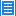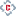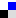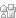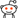您对该条目有什么异议，请填写以下表单，管理员会尽快联系您。 内 容： Email： * 单位： 验证码：刷新
 您在IR的使用过程中有什么好的想法或者建议可以反馈给我们。 标 题： * 内 容： Email： * 验证码：刷新Copyright © 2007-2022  中国科学院软件研究所 - Feedback Powered by CSpace